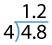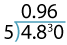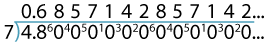Division of decimals

Division of decimals by whole numbers

If we had a piece of rope that was 4.8 metres long and wanted to cut it into four equal pieces we could cut it in two, and then cut each piece in two again to get four pieces. Each piece would be 1.2 metres in length. We can use the division algorithm to show this.The procedure is the same as for whole numbers. The decimal point in the answer is aligned directly above the decimal point in the question.

We can relate this to division by fractions (invert and multiply):
\begin{align}4.8÷4&=\dfrac{48}{10}÷\dfrac{4}{1}\\\\ &=\dfrac{48}{10}×\dfrac{1}{4}\\\\ &=\dfrac{12}{10}\\\\ &=1.2\end{align}

If we were to divide a 4.8-metre piece of rope into five equal pieces, the procedure is a little more involved. We could divide 4.8 by 10 and double the result to get 0.96. This can be also be found using the division algorithm:So, 4.8 cut into five equal lengths is 0.96. Each piece of the rope will be 0.96 metres  in length.

Now, if we divide a 4.8-metre length of rope into seven equal pieces, the result cannot be expressed as a terminating decimal. This can be shown using division:Thus the result of $$4.8 ÷ 7\ \text{is}\ 0.6\dot{8}5714\dot{2}$$, a recurring decimal where the block of digits that recurs is indicated by a dot over the first and last digits of the block. It should be noted that in a practical situation you would probably want to know the length of each piece of rope to the nearest millimetre. Each piece of rope is to be 0.686 metres or 686 millimetres in length, correct to the nearest millimetre.